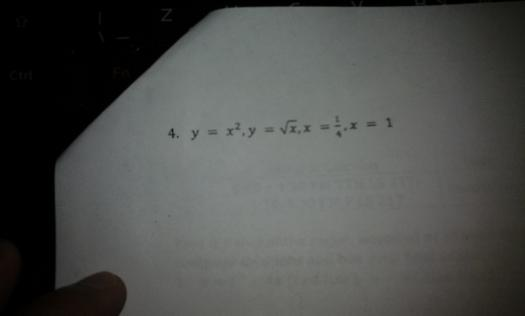# area by integration## moment of inertia of an area with respect to the line x = 2

The moment of inertia bounded by y=x^3 , y=2x , X + y= 6, with respect to x=2 pa help po until Friday. Plus ask ko Lang po ang pinakakapakipakinabang na calculus book for integration applications. Thanks.## area of the region bounded## patulong naman po kung pano isolve 2.

plane areas

Find the area bounded by the curve y = ln x/x, the x-axis and the maximum ordinate.

ans. 1/2

## MATHalino 2020 (Ito Yung Last sa Paatras) Solid mensuration problem

1)An excavation is 12 ft. deep and has trapezoidal sides (faces). The upper base is horizontal rectangle 400 ft. by 180 ft., and the lower base is a horizontal rectangle 350 ft. by 150 ft. How many cubic yards of earth were removed in digging the excavation?